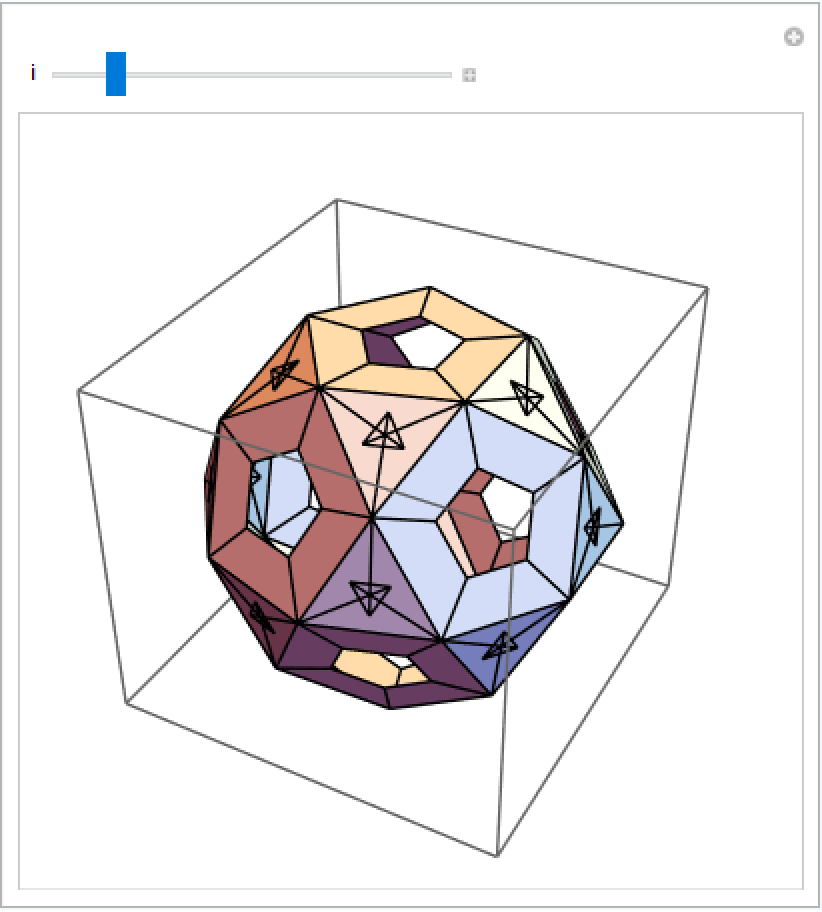#Function Repository Resource:

# RibbonPolyhedron

Display the set of polygons obtained by extending polyhedron edges perpendicularly inward by a given distance

Contributed by: Eric Weisstein
 ResourceFunction["RibbonPolyhedron"][poly,d] shows the ribbon polyhedron obtained by extending edges of polyhedron poly by the distance d. ResourceFunction["RibbonPolyhedron"][poly] uses a default distance of 0.2.

## Details and Options

A ribbon polyhedron is defined by the set of polygons obtained when polyhedron edges are extended perpendicularly inward by a fixed distance.

## Examples

### Basic Examples (1)

"Ribbonize" the cube:

 In:=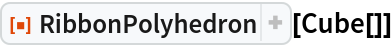Out=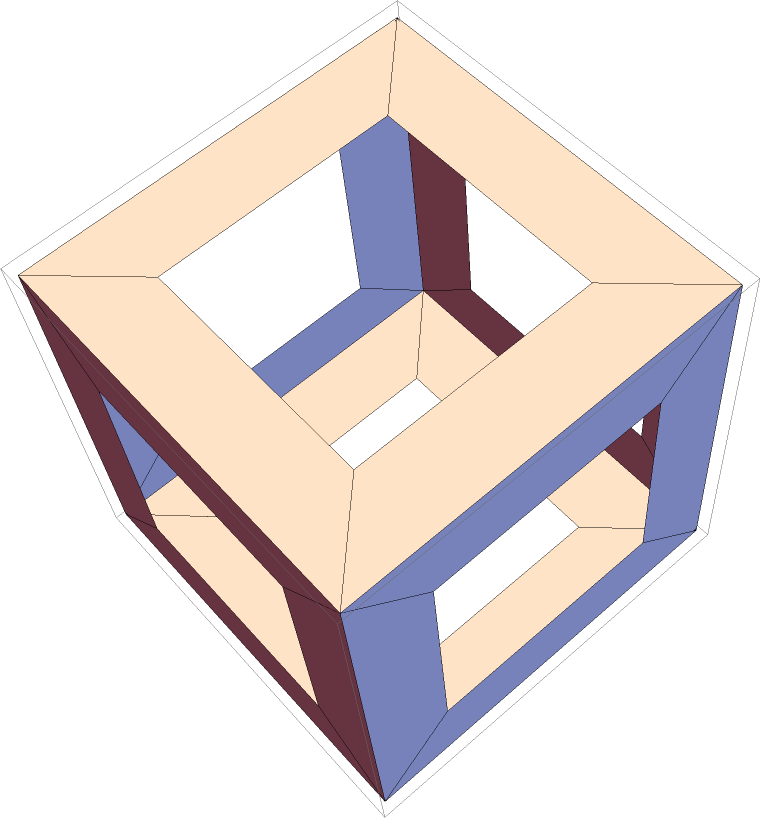### Scope (3)

RibbonPolyhedron works on polyhedron objects:

 In:=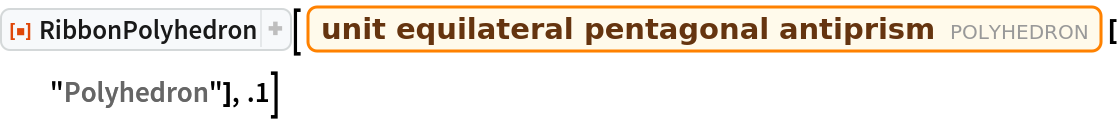Out=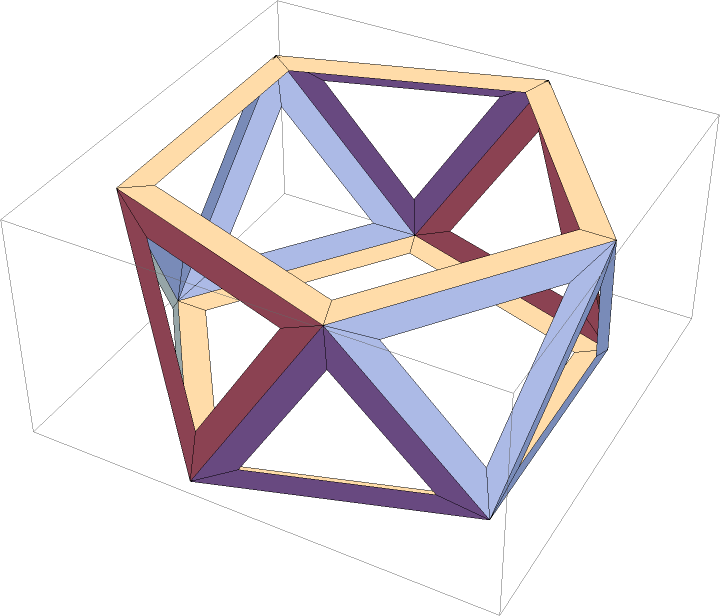RibbonPolyhedron works on polyhedron constructors:

 In:=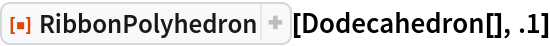Out=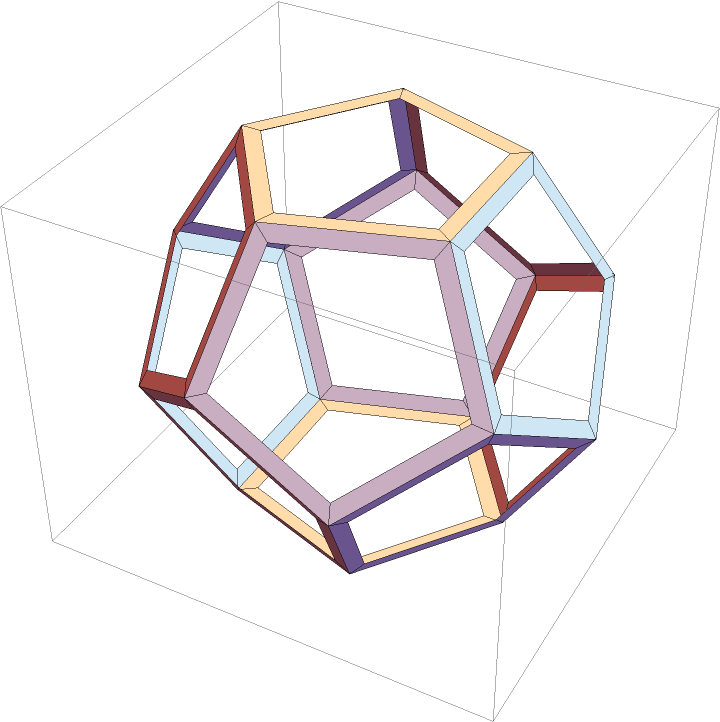Specifying the distance d changes the width of the "ribbons":

 In:=Out=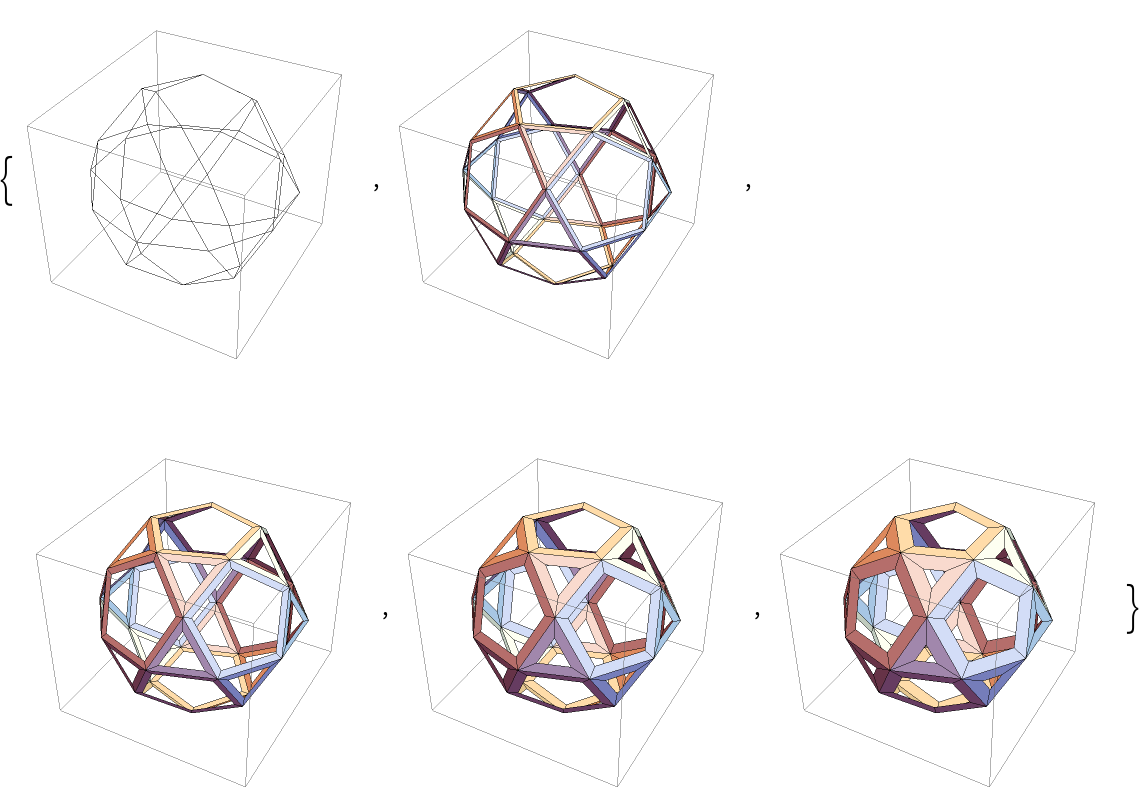### Possible Issues (2)

Large distances may create ribbons that lie outside the original polyhedron:

 In:=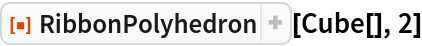Out=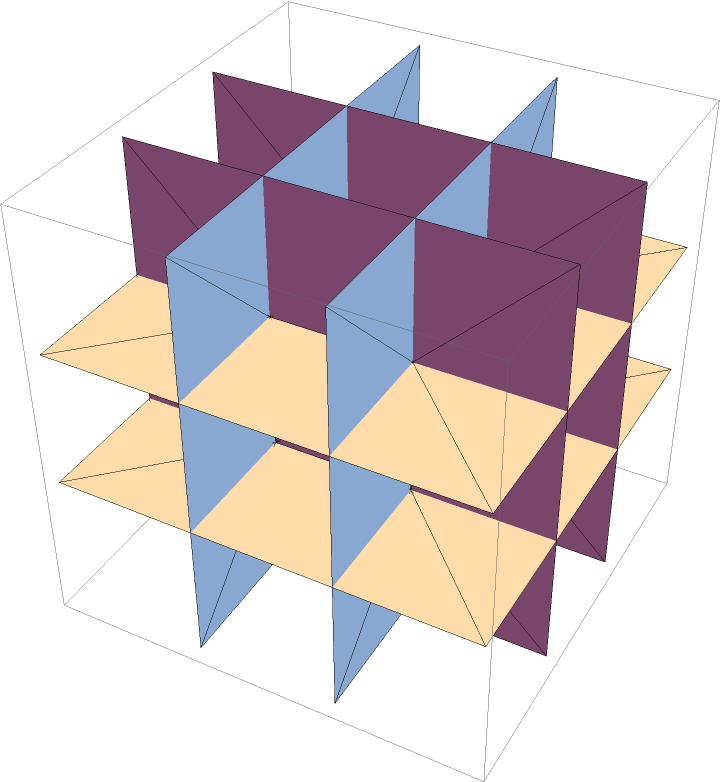For polyhedra with different face types, some ribbons may self-intersect while others do not:

 In:=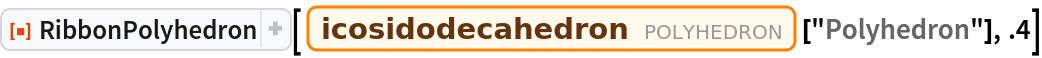Out=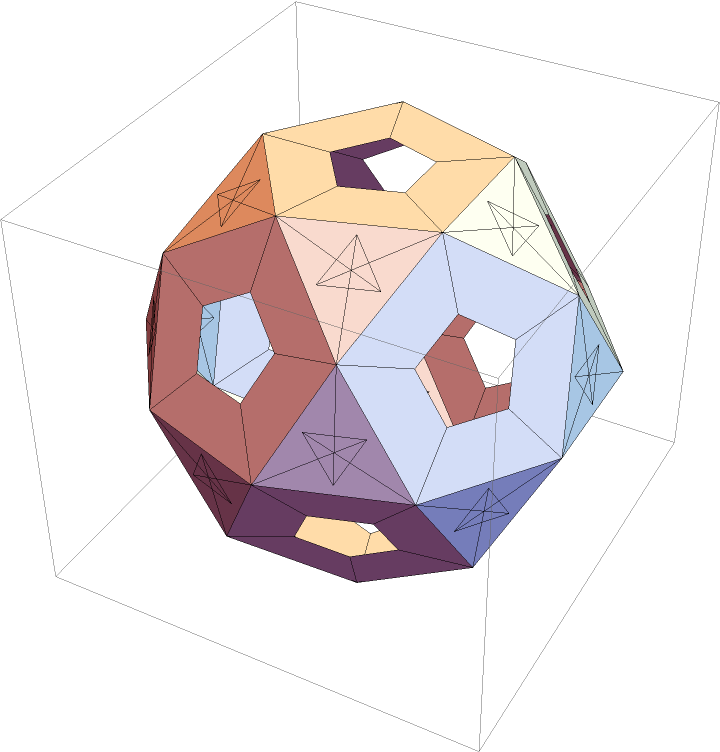### Neat Examples (1)

Explore the "ribbonization" of the icosidodecahedron:

 In:=Out=